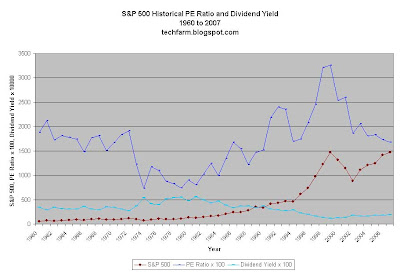## Sunday, February 3, 2008

### Historical S&P 500 PE Ratio and Dividend Yield 1960 to 2007Interpreting the Chart

The Chart above puts three different metrics from 1960 to 2007:
1. The S&P 500 (SPX) value.
2. The PE Ratio of the S&P 500 multiplied by 100. (2007's PE Ratio x 100 in the chart above is 1677.8 which translates to a PE Ratio of 16.8)
3. The Dividend Yield of the S&P 500 multiplied by 100. (2007's Dividend Yield x 100 in the chart above is 189, or 1.89%)

Notes

1. According to wikipedia, the average US equity PE Ratio from 1900 to 2005 is 14 (or 16 depending on how you calculate it). The 2007 PE Ratio of 16.8 is within range of the historical norm.
2. The Bear Market from 1972 to 1974 caused the S&P 500 to go down from around 118 to 68.5 for a decrease of around 42%. The S&P 500 during that time had its PE ratio go from 19 to 7 and the Dividend Yield increase from 2.7% to 5.4% (the dividend yield high between 1960 and 2007)
3. During the Bubble ending around 2001, the S&P 500 PE Ratio reached a very high 32.6 in 1999. The Dividend Yield of the S&P 500 reached a low of 1.14% in 1999.
4. The ensuing Bear Market after the Bubble corrected the stock market and the S&P 500 PE Ratio to a more reasonable PE of 16.8 today.
5. Since 1974, the Dividend Yield has been decreasing steadily to 2007's Dividend Yield of 1.89% (hitting a bottom of around 1.14% in 1999).
6. There appears to be an inverse relationship between Dividend Yield and PE Ratio.

Credits

Research done by Techfarm.blogspot.com and some data taken from Aswath Damoradaran at the New York Stern School of Business.

Unknown said...

It appears you are using Forward P/E which uses operating earnings rather than as-reported earnings to calculate P/E.

Consider making the same chart using as-reported earnings to calculate P/E and see a significant difference in your chart.

techfarmer said...

Good point.

There are a lot of things I can research, but what you suggest above would be an interesting angle on the subject.

 Enter your search terms Submit search form